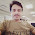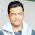DS and Algorithms in Java

In this article, we will learn in details about the Binary Search algorithm.

Let's first we will discuss some basics like what is searching for ? and why do we need searching ?.

What is Searching?

In computer science, searching is the process of finding an item with specified properties from a collection of items. The items may be stored as records in a database, simple data elements in arrays, text in files, nodes in trees, vertices and edges in graphs, or they may be elements of other search spaces.

Why do we need Searching?

Searching is one of the core computer science algorithms. We know that today’s computers store a lot of information. To retrieve this information proficiently we need very efficient searching algorithms.
There are certain ways of organizing the data that improves the searching process. That means, if we keep the data in proper order, it is easy to search the required element. Sorting is one of the techniques for making the elements ordered. In this article, we will see different searching algorithms.

Binary Search with Example

Binary search is a fast search algorithm with run-time complexity of Ο(log n). This search algorithm works on the principle of divide and conquer. For this algorithm to work properly, the data collection should be in the sorted form.
Let us consider the problem of searching for a word in a dictionary. Typically, we directly go to some approximate page [say, middle page] and start searching from that point. If the name that we are searching for is the same then the search is complete. If the page is before the selected pages then apply the same process for the first half; otherwise, apply the same process to the second half. Binary search also works in the same way. The algorithm applying such a strategy is referred to as a binary search algorithm.
Remember – the key aspect here is that the array is already sorted.

How Binary Search Works?

For a binary search to work, it is mandatory for the target array to be sorted. We shall learn the process of binary search with a pictorial example.
The following is our sorted array and let us assume that we need to search the location of value 10 using binary search.
First, we shall determine half of the array by using this formula −
mid = low + (high - low) / 2
Here it is, 0 + (9 - 0 ) / 2 = 4 (integer value of 4.5). So, 4 is mid of the array.
Now we compare the value stored at location 4, with the value being searched, i.e. 10. We find that the value at location 4 is 8, which is not a match. As the value is greater than 8 and we have a sorted array, so we also know that the target value must be in the upper portion of the array.
We change our low to mid + 1 and find the new mid value again.
low = mid + 1
mid = low + (high - low) / 2
Our new mid is 7 now. We compare the value stored at location 7 with our target value 10.
The value stored at location 7 is not a match, rather it is more than what we are looking for. So, the value must be in the lower part from this location.
Hence, we calculate the mid again. This time it is index 5.
We compare the value stored at location 5 with our target value. We find that it is a match.
I conclude that the target value 10 is stored at location 5.

Binary Search Implementation using Java

Let's write a source code for binary search in Java. There are many ways we can write logic for binary search:
1. Iterative implementation
2. Recursive Implementation
3. Using Arrays.binarySearch()
4. Using Collections.binarySearch()

public class BinarySearch { public int binarySearchIteratively(int[] sortedArray, int key) { int low = 0; int high = sortedArray.length - 1; int index = Integer.MAX_VALUE; while (low <= high) { int mid = (low + high) / 2; if (sortedArray[mid] < key) { low = mid + 1; } else if (sortedArray[mid] > key) { high = mid - 1; } else if (sortedArray[mid] == key) { index = mid; break; } } return index; } public static void main(String[] args) { int[] sortedArray = { 0, 1, 2, 3, 4, 5, 5, 6, 7, 8, 9, 9 }; int key = 6; BinarySearch binSearch = new BinarySearch(); int index = binSearch.binarySearchIteratively(sortedArray, key); System.out.println("Search element found " + key+ " in location index : " + index); } }

Output:
Search element  6 found in location index : 7
The binarySearchIteratively method takes a sortedArraykey & the low & high indexes of the sortedArray as arguments. When the method runs for the first time the low, the first index of the sortedArray, is 0, while the high, the last index of the sortedArray, is equal to its length – 1.
The middle is the middle index of the sortedArray. Now the algorithm runs a while loop comparing the key with the array value of the middle index of the sortedArray.

Now, let’s have a look at a simple, recursive implementation as well: public class BinarySearch { public int binarySearchRecursively(int[] sortedArray, int key, int low, int high) { int middle = (low + high) / 2; if (high < low) { return -1; } if (key == sortedArray[middle]) { return middle; } else if (key < sortedArray[middle]) { return binarySearchRecursively(sortedArray, key, low, middle - 1); } else { return binarySearchRecursively(sortedArray, key, middle + 1, high); } } public static void main(String[] args) { int[] sortedArray = { 0, 1, 2, 3, 4, 5, 5, 6, 7, 8, 9, 9 }; int key = 6; BinarySearch binSearch = new BinarySearch(); int index = binSearch.binarySearchRecursively(sortedArray, key, 0, sortedArray.length -1); System.out.println("Search element found in location index : " + index); } }

Output:
Search element found in location index : 7
The binarySearchRecursively method accepts a sortedArraykey, the low and high indexes of the sortedArray.

public class BinarySearch { public int runBinarySearchUsingJavaArrays(int[] sortedArray, Integer key) { int index = Arrays.binarySearch(sortedArray, key); return index; } public static void main(String[] args) { int[] sortedArray = { 0, 1, 2, 3, 4, 5, 5, 6, 7, 8, 9, 9 }; int key = 6; BinarySearch binSearch = new BinarySearch(); int index1 = binSearch.runBinarySearchUsingJavaArrays(sortedArray, key); System.out.println("Search element found in location index : " + index1); } }

Output:
Search element found in location index : 7

public class BinarySearch { public int runBinarySearchUsingJavaCollections(List<Integer> sortedList, Integer key) { int index = Collections.binarySearch(sortedList, key); return index; } public static void main(String[] args) { Integer[] sortedArray = { 0, 1, 2, 3, 4, 5, 5, 6, 7, 8, 9, 9 }; int key = 6; BinarySearch binarySearch = new BinarySearch(); int index1 = binarySearch.runBinarySearchUsingJavaCollections(Arrays.asList(sortedArray), key); System.out.println("Search element found in location index : " + index1); } }

Output:
Search element found in location index : 7
Time Complexity: The time complexity of Binary Search can be written as
T(n) = T(n/2) + c
Auxiliary Space: O(1) in the case of iterative implementation. In case of recursive implementation, O(Logn) recursion call stack space.
Algorithmic Paradigm: Decrease and Conquer.

DS and Algorithms in Java

1.1.